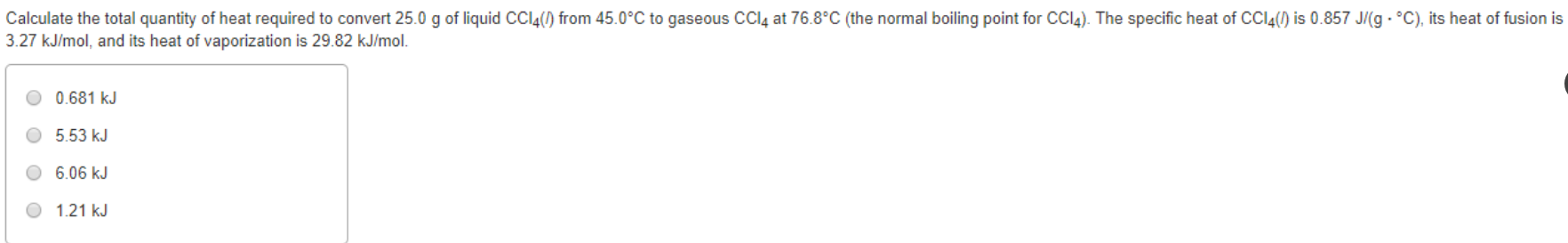# Calculate the total quantity of heat required to convert 25.0 g of liquid CCl4(l) from 45.0 degrees C to gaseous CCl4 at 76.8 degrees C (the normal boiling point of CCl4). The specific heat of CCl4(l) is 0.857 J/(g . degrees C), its heat of fusion is 3.27 kJ/mol, and its heat of vaporization is 29.82 kJ/mol a) 0.681 kJ b) 5.53 kJ c) 6.06 kJ d) 1.21 kJ